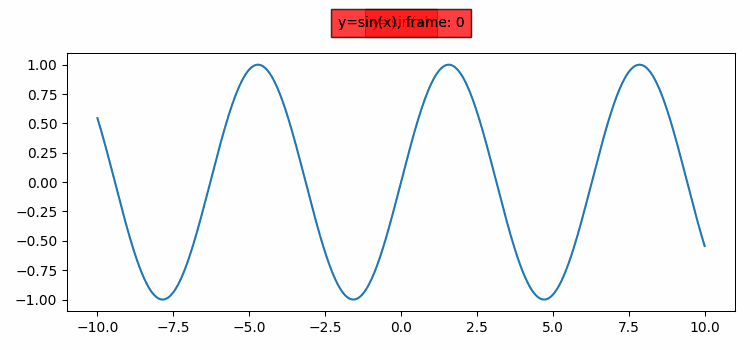# How to update the plot title with Matplotlib using animation?

To update the plot title with Matplotlib using animation, we can take the following steps −

• Set the figure size and adjust the padding between and around the subplots.
• Create a new figure or activate an existing figure using figure() method.
• Create x and y data points using numpy.
• Get the current axis.
• Add text to the axes using text() method.
• Add an animate method that can be used to make an animation by repeatedly calling a function.
• To display the figure, use show() method.

## Example

import numpy as np
from matplotlib import pyplot as plt, animation

plt.rcParams["figure.figsize"] = [7.50, 3.50]
plt.rcParams["figure.autolayout"] = True

fig = plt.figure()

x = np.linspace(-10, 10, 1000)
y = np.sin(x)

plt.plot(x, y)

ax = plt.gca()
ax.text(0.5, 1.100, "y=sin(x)", bbox={'facecolor': 'red',
transform=ax.transAxes, ha="center")

def animate(frame):
ax.text(0.5, 1.100, "y=sin(x), frame: %d" % frame,
bbox={'facecolor': 'red', 'alpha': 0.5, 'pad': 5},
transform=ax.transAxes, ha="center")

ani = animation.FuncAnimation(fig, animate, interval=100,
frames=10, repeat=True)

plt.show()

## Output